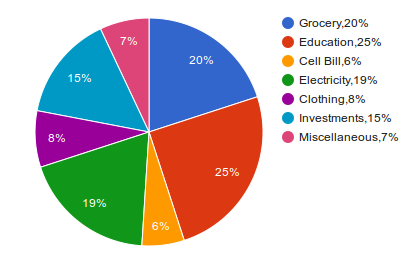# IBPS RRB PO 2017

Instructions

What should come in place of the question mark (?) in the following question ?

Question 61

Question 62

Question 63

Question 64

Question 65

# $$\sqrt{21952}+33=?$$

Instructions

Study the following Pie Graph carefully and answer the questions given below:

The pie chart given below represents the budget estimated by a family for their monthly expenses. The total salary is Rs. 32,000 per monthQuestion 66

Question 67

Question 68

Question 69

Question 70

OR